# Simple Interest Worksheets for Class 5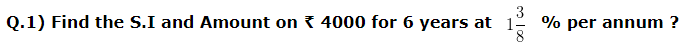a) Simple Interest = ₹ 260 , Amount = ₹ 4430
b) Simple Interest = ₹ 330 , Amount = ₹ 4330
c) Simple Interest = ₹ 249 , Amount = ₹ 4260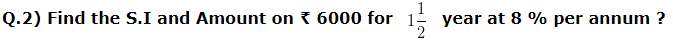a) Simple Interest = ₹ 842 , Amount = ₹ 6795
b) Simple Interest = ₹ 790 , Amount = ₹ 6570
c) Simple Interest = ₹ 720 , Amount = ₹ 6720

Q.3) Find the S.I and Amount on ₹ 5500 for 2 year at 3 % per annum ?
a) Simple Interest = ₹ 330 , Amount = ₹ 5830
b) Simple Interest = ₹ 230 , Amount = ₹ 5840
c) Simple Interest = ₹ 280 , Amount = ₹ 6130

Q.4) Rohan borrowed ₹ 15500 from a bank for 2 years at the rate of 3 % per annum. How much interest will he be charged ?
a) ₹ 1130
b) ₹ 930
c) ₹ 1430

### Simple Interest Worksheets for Class 5 Explanations

Q.1) Explanation – Simple Interest Worksheets for Class 5

Principle(P) = Money Borrowed from others or lent to others or invested is called the Principal.
Rate (R) = Percentage , at which interest is charged or received
Time(T) = Time period for which the money is borrowed or lent.
Simple interest (S.I) = Extra money received or paid on principal
Given
Principle(P) = ₹ 4000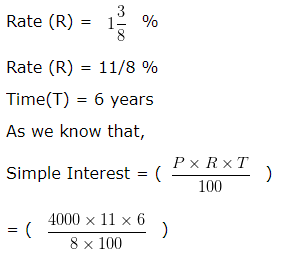(When rate of interest is a fraction, the numerator is written in numerator and the denominator is written in denominator in the formula of S.I )
= 2640/8
= ₹ 330
Hence, Simple Interest = ₹ 330
We know that
Amount = Principle(P) + Simple Interest
Amount = 4000 + 330
= ₹ 4330
So, the Simple Interest is ₹ 330 and the Amount is ₹ 4330

Correct Answer – b) Simple Interest = ₹ 330 , Amount = ₹ 4330

Q.2) Explanation – Simple Interest Worksheets for Class 5

Principle(P) = Money Borrowed from others or lent to others or invested is called the Principal.
Rate (R) = Percentage , at which interest is charged or received
Time(T) = Time period for which the money is borrowed or lent.
Simple interest (S.I) = Extra money received or paid on principal
Given
Principle(P) = ₹ 6000
Rate (R) = 8 %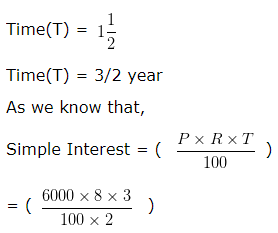= 1440/2
= ₹ 720
Hence, Simple Interest = ₹ 720
We know that
Amount = Principle(P) + Simple Interest
Amount = 6000 + 720
= ₹ 6720
So, the Simple Interest is ₹ 720 and the Amount is ₹ 6720

Correct Answer – c) Simple Interest = ₹ 720 , Amount = ₹ 6720

Q.3) Explanation – Simple Interest Worksheets for Class 5

Principle(P) = Money Borrowed from others or lent to others or invested is called the Principal.
Rate (R) = Percentage , at which interest is charged or received
Time(T) = Time period for which the money is borrowed or lent.
Simple interest (S.I) = Extra money received or paid on principal
Given
Principle(P) = ₹ 5500
Rate (R) = 3 %
Time(T) = 2 year
As we know that,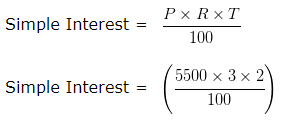Simple Interest = ₹ 33000/100
Simple Interest = ₹ 330
Hence, Simple Interest = ₹ 330
We know that
Amount = Principle(P) + Simple Interest
Amount = 5500 + 330
Amount = ₹ 5830
So, the Simple Interest is ₹ 330 and the Amount is ₹ 5830

Correct Answer – a) Simple Interest = ₹ 330 , Amount = ₹ 5830

Q.4) Explanation – Simple Interest Worksheets for Class 5

Money borrowed by Rohan = ₹ 15500
Principle(P) = Money Borrowed from others or lent to others is called the Principal.
Principle(P) = ₹ 15500
Rate (R) = 3 %
Time(T) = 2 years
As we know that,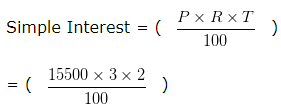= 93000/100
= ₹ 930
So, interest paid by Rohan = ₹ 930

Correct Answer – b) ₹ 930